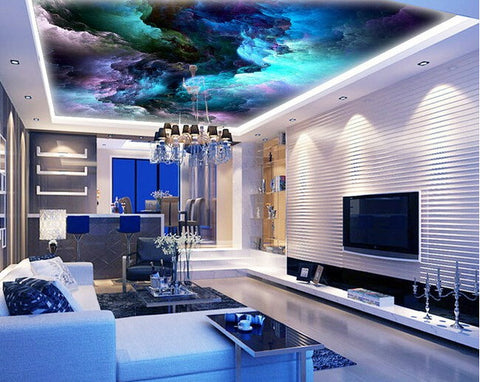## Modern Abstract Blue and Black Sky Swirling Clouds Ceiling Mural

• \$39.95

Product Details

• Function: Waterproof, Smoke-Proof, Moisture-Proof, Mold Proof, Soundproof, Sound-Absorbing, Heat Insulation, Anti-static
• Material: 3D relief, Waterproof oil canvas, Silk cloth

IMPORTANT: PLEASE READ!  Minimum order is 2 square meters (2 pieces) \$79.90.  This will NOT cover a full wall. To cover a complete wall you will need more.  The example pictures show a wall that is fully covered with wallpaper.  You need to measure your wall and calculate how many pieces you need.  Or you can choose from one of the fixed sizes below.  When you check-out, select "add to cart".  Then choose the quantity you need.

How to calculate how many pieces you need:
1). Measure your wall.  Convert your measurements to centimeters (you can use any online metric converter). 2). If your wall is 360cm width x 240cm height for example, multiply those numbers: 360 x 240 = 8.6.  So you would need to buy 9 pieces.
Or, if you prefer, you can choose one of our fixed sizes:

Quantity 2  :  2 square meter = 200cm(W) x 100cm(H) (6'7" x 3'3") \$79.90

Quantity 3  :  3 square meter = 220cm(W) x 140cm(H) (7'3" x 4'7") \$119.85

Quantity 4  :  4 square meter = 250cm(W) x 160cm(H) (8'2" x 5'3") \$159.80

Quantity 5  :  5 square meter = 280cm(W) x 180cm(H) (9'2" x 5'11") \$199.75

Quantity 6  :  6 square meter = 300cm(W) x 200cm(H) (9'10" x 6'7") \$239.70

Quantity 7  :  7 square meter = 330cm(W) x 210cm(H) (10'10" x 6'11") \$279.65

Quantity 8  :  8 square meter = 360cm(W) x 230cm(H) (11'10" x 7'6") \$319.60

Quantity 9  :  9 square meter = 380cm(W) x 240cm(H) (12'5" x 7'10") \$359.55

Quantity 10 :  10 square meter = 400cm(W) x 250cm(H) (13'1" x 8'2") \$399.50

Quantity 11 : 11 square meter = 420cm(W) x 260cm(H) (13'9'' x 8'6'') \$439.45

Quantity 12 : 12 square meter = 440cm(W) x 270cm(H) (14'5" x 8'10") \$479.40

Quantity 13 : 13 square meter = 460cm(W) x 280cm(H) (15'1'' x 9'2'') \$519.35

Quantity 14 : 14 square meter = 480cm(W) x 290cm(H) (15'9'' x 9'6'') \$559.30

Quantity 15 : 15 square meter= 500cm(W) x 300cm(H) (16'5'' x 9'10'') \$599.25

Quantity 16 : 16 square meter=500cm(W) x 320cm(H) (16'5" x 10'6") \$639.20

We Also Recommend